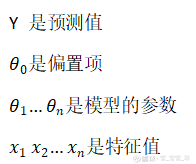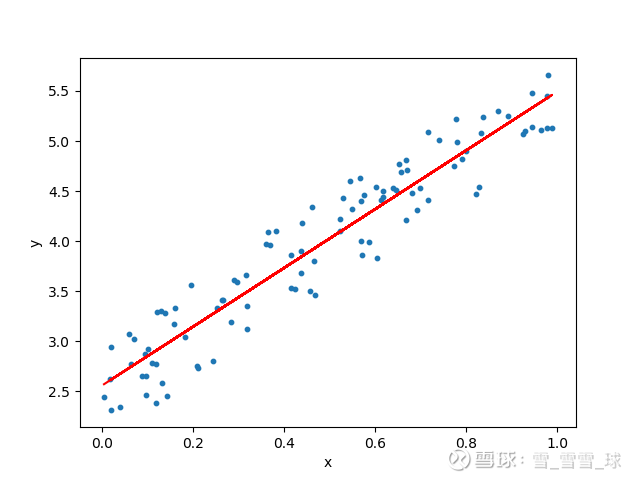1. model.py -- 包含机器学习模型的代码，用于根据前两个月的销售额预测第三个月的销售额。

3. request.py -- 使用requests模块调用app.py中定义的API并显示返回值。

4. HTML/CSS – 包含HTML模板和CSS风格代码，允许用户输入销售细节并显示第三个月的预测值。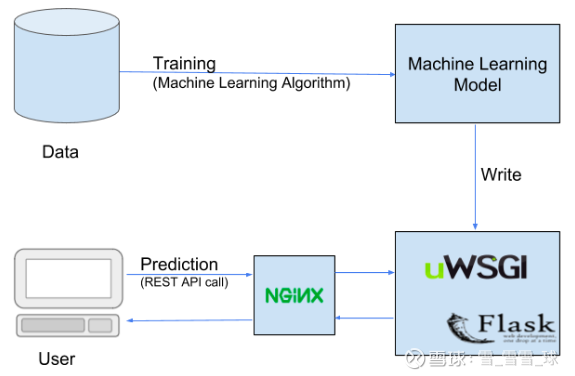1. Scikit-learn

2. Pandas

3. Numpy

@import url(网页链接);
html { width: 100%; height:100%; overflow:hidden;
}body {width: 100%;height:100%;font-family: 'Helvetica';background: #000;
color: #fff;
font-size: 24px;
text-align:center;
top: 40%;
left: 50%;
margin: -150px 0 0 -150px;
width:400px;
height:400px;}

letter-spacing:1px;
text-align:center;
}input {width: 100%;
margin-bottom: 10px;
background: rgba(0,0,0,0.3);
border: none;
outline: none;
font-size: 13px;
color: #fff;
border: 1px solid rgba(0,0,0,0.3);
box-shadow: inset 0 -5px 45px rgba(100,100,100,0.2), 0 1px 1px rgba(255,255,255,0.2);
}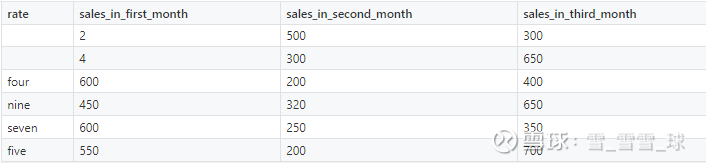import numpy as np
import matplotlib.pyplot as plt
import pandas as pd
import pickle
dataset['rate'].fillna(0, inplace=True)
dataset['sales_in_first_month'].fillna(dataset['sales_in_first_month'].mean(), inplace=True)
X = dataset.iloc[:, :3]
def convert_to_int(word):
word_dict = {'one':1, 'two':2, 'three':3, 'four':4, 'five':5, 'six':6, 'seven':7, 'eight':8,
'nine':9, 'ten':10, 'eleven':11, 'twelve':12, 'zero':0, 0: 0}
return word_dict[word]
X['rate'] = X['rate'].apply(lambda x : convert_to_int(x))
y = dataset.iloc[:, -1]
from sklearn.linear_model import LinearRegression
regressor = LinearRegression()
regressor.fit(X, y)
pickle.dump(regressor, open('model.pkl','wb'))
print(model.predict([[4, 300, 500]]))

import numpy as np
import pickle
@app.route('/')
def home():
return render_template('index.html')
@app.route('/predict',methods=['POST'])
def predict():
int_features = [int(x) for x in request.form.values()]
final_features = [np.array(int_features)]
prediction = model.predict(final_features)
output = round(prediction, 2)
return render_template('index.html', prediction_text='Sales should
be \$ {}'.format(output))
@app.route('/results',methods=['POST'])
def results():
data = request.get_json(force=True)
prediction = model.predict([np.array(list(data.values()))])
output = prediction
return jsonify(output)
if __name__ == "__main__":
app.run(debug=True)

import requests
url = 'http://localhost:5000/results'
r = requests.post(url,json={'rate':5,
'sales_in_first_month':200, 'sales_in_second_month':400})
print(r.json()) Results

python app.py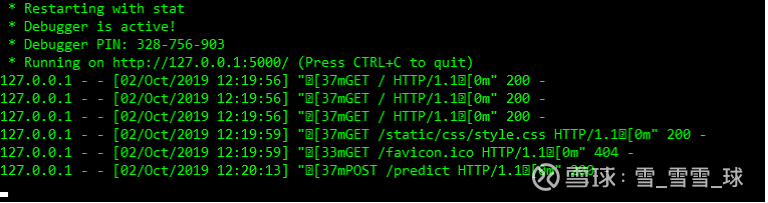How to Easily Deploy Machine Learning Models Using Flask

▼▼▼（*本文为AI科技大本营转载文章，转载联系原作者

2019 中国大数据技术大会（BDTC）再度来袭！豪华主席阵容及百位技术专家齐聚，15 场精选专题技术和行业论坛，超强干货+技术剖析+行业实践立体解读，深入解析热门技术在行业中的实践落地。6.6 折票限时特惠（立减1400元），学生票仅 599 元！ICCV 2019 | 无需数据集的Student Networks

2019年不可错过的45个AI开源工具，你想要的都在这里

“老赖”罗永浩：就算“卖艺”也会还债，孙宇晨：我买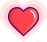11-04 14:53 雪球 转发：0 回复：0 喜欢：2